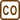## Ideal Gas Versus Van der Waals

### Task number: 1284

A steel bomb with a volume of 0.53 m3 is filled with carbon dioxide with the amount of substance 1 kmol and the pressure of 5.07 MPa. What is the difference between the temperature of the gas calculated by using the model of an ideal gas and the temperature calculated by using the Van der Waals equation?

• #### Numerical values

 V = 0.53 m3 the internal volume of the container n = 1 kmol = 103 mol the amount of substance of carbon dioxide p = 5.07 MPa = 5.07·106 Pa the pressure of carbon dioxide ΔT = ? the difference between the temperatures calculated by each of the models

From The Handbook of Chemistry and Physics:

 R = 8.31 Jmol−1K−1 the molar gas constant a = 0.365 Jm3mol−2 the "van der Waals" constant for carbon dioxide b = 4.28·10−5 m3mol−1 the "van der Waals" constant for carbon dioxide
• #### Analysis

We will evaluate the thermodynamic temperature from both the ideal gas equation and the Van der Waals equation and then we will calculate their difference.

• #### Solution

First we evaluate the thermodynamic temperature Ti from the ideal gas equation

$pV=nRT_{i},$

where p is the pressure, V is the volume, n is the amount of substance of carbon dioxide and R is the molar gas constant.

$T_{i}=\frac{pV}{nR}.$

We also evaluate the thermodynamic temperature Tv from the van der Waals equation

$\left(p+\frac{n^{2}a}{V^{2}}\right)(V-nb)=nRT_{v},$

where a and b are the van der Waals constants for carbon dioxide, and thus we obtain

$T_{v}=\frac{\left(p+\frac{n^{2}a}{V^{2}}\right)(V-nb)}{nR}.$

Finally, we determine the temperature difference ΔT:

$\mathrm{\Delta}T=T_{v}-T_{i}=\frac{\left(p+\frac{n^{2}a}{V^{2}}\right)(V-nb)-pV}{nR}.$
• #### Numerical insertion

$\mathrm{\Delta}T=\frac{\left(p+\frac{n^{2}a}{V^{2}}\right)(V-nb)-pV}{nR}$ $\mathrm{\Delta}T=\frac{\left(5.07\cdot{10^6}+\frac{10^6\cdot{0.365}}{0.53^{2}}\right)(0.53-10^3\cdot{4.28}\cdot{10^{-5}})-5.07\cdot{10^6}\cdot{0.53}}{8.31\cdot{10^3}}\,\mathrm{K}$ $\mathrm{\Delta}T\dot{=}50\,\mathrm{K}$
• #### Answer

The temperature calculated by the van der Waals equation is approximately 50 K higher than the temperature calculated by the ideal gas equation.